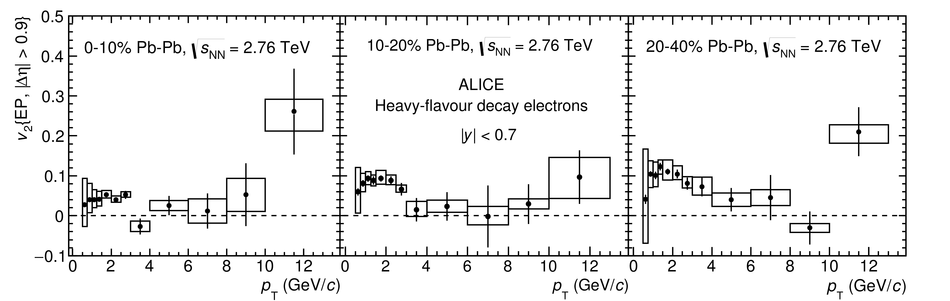# Figure 8

 Elliptic flow of electrons from heavy-flavour hadron decays in the 0-10% (left panel), 10-20% (middle panel) and 20-40% (right panel) centrality classes in Pb-Pb collisions at $\sqrt{s_{\rm NN}}$ = 2.76 TeV at mid-rapidity as function of $p_{\rm T}$. The symbols are placed at the centre of the $p_{\rm T}$ interval whose width is shown by the horizontal error bar. The vertical error bars and open boxes represent the statistical and systematic uncertainties, respectively. The results are obtained with the event plane method and an eta gap $|\Delta \eta|$ $>$ 0.9.